QuestionChapter 13, Problem 118AP
Interpretation Introduction

Expert Solution

## Answer to Problem 118AP

The monatomic gas is Argon.

### Explanation of Solution

The ideal gas equation is

PV=nRT

Where,

P = Pressure of the gas = 1.00 atm

V = Volume of the gas = 2.50 L

n = moles of the gas = ?

R = Universal gas constant = 0.0821 L.atm/mol.K

T = Temperature of the gas = -48 ° C = 225 K

Substituting the values in the given equation, we get,

PV=nRT1.00atm×2.50L=n×0.0821L.atm/mol.K×225Kn=0.135mol

Thus, the moles of the gas = 0.135 mol

From the moles of the gas, one can find the molar mass of the gas thereby identity of the gas.

Moles=MassMolar mass0.135mol=5.41gMM=40.074g/mol

The monatomic gas with this molecular weight is Argon.

Interpretation Introduction

Expert Solution

## Answer to Problem 118AP

Temperature of gas mixture = 225 K

Total moles of gas mixture = 0.447 mol

Total pressure of gas mixture = 1 atm

Volume of balloon = 8.26 L.

### Explanation of Solution

Given, 10.0 g of oxygen is added.

Moles of oxygen are to be found.

Moles=MassMolar massMoles=10.0g32g/mol=0.3125 mol

Moles of oxygen = 0.3125 mol

Moles of monatomic gas = 0.135 mol

Total number of moles = 0.3125 mol + 0.135 mol = 0.447 mol

Air inside the balloon and atmospheric air pressure has very small pressure difference.

Therefore, one can consider it same and assume here that pressure of air inside balloon is equal to atmospheric pressure that is 1 atm.

Since, there is no change in temperature so, the temperature of the mixture is 225 K.

Total volume of gas mixture is found using ideal gas equation.

PV=nRT1atm×V=0.447mol×0.0821L.atm/mol.K×225KV=8.26L

Thus,

Temperature of gas mixture = 225 K

Total moles of gas mixture = 0.447 mol

Total pressure of gas mixture = 1 atm

Volume of balloon = 8.26 L.

Interpretation Introduction

Expert Solution

## Answer to Problem 118AP

Temperature of gas mixture = 225 K

Total moles of gas mixture = 0.447 mol

Total pressure of gas mixture = 3.303 atm

Volume of rigid container = 2.5 L.

### Explanation of Solution

Given, 10.0 g of oxygen is added.

Moles of oxygen are to be found.

Moles=MassMolar massMoles=10.0g32g/mol=0.3125 mol

Moles of oxygen = 0.3125 mol

Moles of monatomic gas = 0.135 mol

Total number of moles = 0.3125 mol + 0.135 mol = 0.447 mol

Since, there is no change in temperature so, the temperature of the mixture is 225 K.

Since, the given container is rigid so, the volume of the mixture is 2.50 L.

Total pressure of gas mixture is found using ideal gas equation.

PV=nRTP×2.50L=0.447×0.0821L.atm/mol.K×225KP=3.303atm

Thus,

Temperature of gas mixture = 225 K

Total moles of gas mixture = 0.447 mol

Total pressure of gas mixture = 3.303 atm

Volume of rigid container = 2.5 L.

### Want to see more full solutions like this?

Subscribe now to access step-by-step solutions to millions of textbook problems written by subject matter experts!Not helpful? See similar booksWant to see more full solutions like this?
Subscribe now to access step-by-step solutions to millions of textbook problems written by subject matter experts.
Knowledge BoosterNeed a deep-dive on the concept behind this application? Look no further. Learn more about this topic, chemistry and related others by exploring similar questions and additional content below.
Need a deep-dive on the concept behind this application? Watch these videos for an in-depth look
Recommended textbooks for you
•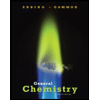General Chemistry - Standalone book (MindTap Cour...
Chemistry
ISBN:9781305580343
Author:Steven D. Gammon, Ebbing, Darrell Ebbing, Steven D., Darrell; Gammon, Darrell Ebbing; Steven D. Gammon, Darrell D.; Gammon, Ebbing; Steven D. Gammon; Darrell
Publisher:Cengage Learning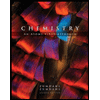Chemistry: An Atoms First Approach
Chemistry
ISBN:9781305079243
Author:Steven S. Zumdahl, Susan A. Zumdahl
Publisher:Cengage Learning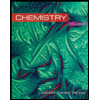Chemistry
Chemistry
ISBN:9781305957404
Author:Steven S. Zumdahl, Susan A. Zumdahl, Donald J. DeCoste
Publisher:Cengage Learning
•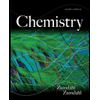Chemistry
Chemistry
ISBN:9781133611097
Author:Steven S. Zumdahl
Publisher:Cengage Learning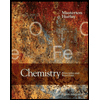Chemistry: Principles and Reactions
Chemistry
ISBN:9781305079373
Author:William L. Masterton, Cecile N. Hurley
Publisher:Cengage Learning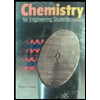Chemistry for Engineering Students
Chemistry
ISBN:9781337398909
Author:Lawrence S. Brown, Tom Holme
Publisher:Cengage Learning
•General Chemistry - Standalone book (MindTap Cour...
Chemistry
ISBN:9781305580343
Author:Steven D. Gammon, Ebbing, Darrell Ebbing, Steven D., Darrell; Gammon, Darrell Ebbing; Steven D. Gammon, Darrell D.; Gammon, Ebbing; Steven D. Gammon; Darrell
Publisher:Cengage LearningChemistry: An Atoms First Approach
Chemistry
ISBN:9781305079243
Author:Steven S. Zumdahl, Susan A. Zumdahl
Publisher:Cengage LearningChemistry
Chemistry
ISBN:9781305957404
Author:Steven S. Zumdahl, Susan A. Zumdahl, Donald J. DeCoste
Publisher:Cengage LearningChemistry
Chemistry
ISBN:9781133611097
Author:Steven S. Zumdahl
Publisher:Cengage LearningChemistry: Principles and Reactions
Chemistry
ISBN:9781305079373
Author:William L. Masterton, Cecile N. Hurley
Publisher:Cengage LearningChemistry for Engineering Students
Chemistry
ISBN:9781337398909
Author:Lawrence S. Brown, Tom Holme
Publisher:Cengage Learning
Step by Step Stoichiometry Practice Problems | How to Pass ChemistryMole Conversions Made Easy: How to Convert Between Grams and Moles; Author: Ketzbook;https://www.youtube.com/watch?v=b2raanVWU6c;License: Standard YouTube License, CC-BY